##### 7 - Atoms & QM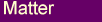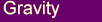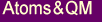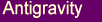Here we look at atomic structure and quantum mechanics from the 4D aetheric wave and fluid dynamics perspective, including the particle wave duality of matter and higher dimensions.

#### Atoms, Aether and 4D Waves

As outlined in the Matter section, the present model assumes nuclear particles to be condensations of aether at points where 4D waves produce nodes of low vibration.
While electrons are assumed to be aether vortexes that connect to the protons via the 4th dimension.
However the electron vortexes are not positioned at nodal points.
In the same way that atmospheric vortexes, such as tornadoes, form in air that is turbulent it would make sense that the electron vortexes would form in regions of high aetheric vibration. That is, regions surrounding the nodes where the 4D wave oscillations are at a maximum.
Thus we have an image of an atom as composed of a nucleus siting at a nodal point surrounded by electron vortexes being continuously moved about in the high vibration regions around the nucleus by aetheric currents.

The above picture would imply that the first peak of aetheric vibration would occur around the 0.5 Angstrom (0.5x10-10m) distance from the nucleus corresponding to the first electron orbital of the Hydrogen atom.
Therefore it would make sense that the shortest 4D wave would be such as to produce a peak at the 0.5 Angstrom distance. In other words, a wavelength of around 1 Angstrom.
It is also expected that there would be waves generated whose wavelengths are multiples of the shortest wavelength. These would be the harmonics of the basic wave that combine in such a way as to produce the required low and high vibration regions surrounding the atoms.

The 4D vibrations would also be expected to create complex aether flows in 3D space in the presence of 'hard' matter such as protons and neutrons.
This is supported by the observation that neutrons outside the nucleus will after a short period spontaneously transform into a electron-proton pair.
This implies that the presence of the neutron changes the surrounding aether in such a way as to promote the formation of the electron-proton pair.
That such an event should occur is difficult to explain conceptually with standard models.
This model might also help us to understand some unusual observations that seem to involve the reverse process of electron-proton pairs being converted into neutrons inside atoms. That is, transmutation of the elements.
There have been reports over the years of processes that produce conversions from one element to another.
One example is the Marcus Hollingshead device, consisting of orthogonal spinning toroidal coils, that over a period of time changes the properties of the materials inside it  . The material was found to have a greater proportion of neutrons than it started out with.
The well known cold fusion experiments are another example.
One mechanism that might be involved in some of these processes is the breaking of an electron-proton 4D vortex connection, perhaps by use of high powered electromagnetic fields oscillation at resonant frequencies, leading to a dissolution of the electron-proton charge vortexes. This would effectively be a conversion of an electron-proton pair into a neutron, without having to force the electrons close enough to the nucleus to merge with the protons.

#### Aether and Quantum Mechanics

The standard quantum mechanical wave equation essentially describes standing waves.
The reasoning being that only standing waves can be used to describe a stable orbit of electrons around the nucleus. Similar to the way a string on a guitar will form standing wave patterns for wavelengths that are multiples of the string length.
The requirement of standing waves is not too different from what is required in the 4D wave aetheric model. Only nodal points that persist for many wave periods will be stationary long enough for nuclear particles to firstly condense out of the aether and secondly for the atoms to travel with the nodes.

The basic quantum mechanical wave equation is of the form: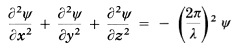The requirement of a standing wave pattern then leads to the condition that only certain wavelengths are allowed in the solution of the above equation.
In the standard theory particles are associated with a wavelength that is proportional to their momentum (p= mv) leading to the quantum nature of atoms. That is, the electrons can have only certain energy values (E) corresponding to the allowed wavelengths through the equality: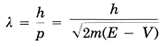However we think that it is not necessary to attribute a wave nature to the particles themselves.
Perhaps we have been looking at this the wrong way?
Perhaps the wave nature is not a component of the particles but of the medium in which they are embedded, the aether.
As outlined above, the 4D wave model assumes that the electrons occupy regions of high aetheric vibration around the nodal points. Therefore in this model the electrons are driven around the nuclear orbits by their interactions with the 4D wave oscillations of the aether.
It may be that the wave function of quantum mechanics, as it applies to atomic structure, corresponds in some way to the level of vibration in the aether.
This is similar to certain models in Quantum Electrodynamics which seek to explain quantum mechanical effects as a consequence of a particle's interaction with the zero-point energy fluctuations .

#### The Problem of the Non-Radiating Electron

One of the reasons that Quantum Mechanics was created in the first place was to try and explain why orbiting electrons inside an atom do not generate continuous electromagnetic radiation as a result of being accelerated around the nucleus, as would normally be the case for an accelerated charge.
One would expect that such an electron would continuously radiate away energy and as a result spiral into the nucleus collapsing the atom.
The quantum mechanical explanation is that the electron is to be described by a standing wave pattern, as described by the wave function, which only forms a stable configuration for certain wavelengths. As discussed in the previous section, this implies that the energy of a particular electron orbital is constant since it is proportional to the wavelength.
Therefore it is argued that an orbital with a constant energy cannot radiate away energy.
This however is an artificial explanation, it does not really explain why the electron cannot radiate.
Even if we accept the idea, as implied by the uncertainty principle, that we cannot in principle know where an electron is inside an atom a localized particle orbiting a nucleus should still radiate energy, even if we do not know where it is.
Otherwise, we have to assume that the electron is some kind of 'smeared out' amorphous substance - a philosophically ugly notion that at the conceptual level creates more problems than it solves.
Standard theory attributes a velocity for the different electron orbitals which for a Hydrogen atom is given by: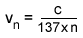where    vn = electron velocity for n orbital
n  = 1,2,3...  the orbital quantum number

We see that for the ground state electron (1s orbital) in Hydrogen the speed is slightly under 1% of the speed of light. The speed decreasing in proportion to 1/n.
The very notion of an orbital velocity implies a localized particle. It is difficult to see how the concept of an orbital velocity could be applied to a 'smeared out' substance as implied by the wave function.
Inherent in Quantum Mechanics are two contradictory pictures of the electron, one a localized particle as implied by the orbital velocity and the potential energy function, the other a 'smeared out' substance as implied by the wave equation.
As already discussed, we believe that it makes more sense to attribute the wave properties to the medium or aether than to the particles themselves, eliminating the contradiction.

However, with this model we still need to explain why the orbiting electrons do not radiate electromagnetic energy and spiral into the nucleus.
As described in the Light page our model assumes that electromagnetic radiation is generated whenever a charge is accelerated relative to the aether.
One way to explain the non-radiation is to assume that the electrons do not accelerate relative to the aether. In other words, it is possible that electrons are carried along on currents of flowing aether, similar to the way vortexes on the surface of rivers are carried along by the flowing water.
This would imply that for each electron orbital there are stable orbiting aether currents along which the electrons move.
Furthermore, when the electron jumps from one orbital to another it would accelerate relative to the aether and would therefore radiate a photon, along the lines discussed on our Light page.

#### Quantum Mechanics or Fluid Dynamics?

The concept of the quantum mechanical wave equation corresponding to vibrations in some gas/liquid medium is further supported by the work of R. M. Kiehn and others who have pointed out the similarities between the wave equation and fluid dynamics.
R. M. Kiehn has shown that there exists a direct mapping between the Schroedinger wave equation for a charged particle in a magnetic field and the Navier-Stokes fluid dynamic equations for vortex motion in a compressible viscous fluid .
The absolute square of the wave function, which normally represents the probability of finding a particle in a given position, is then equal to the vorticity distribution in the fluid.
The mapping between the two systems is complete with the following equality for the kinematic viscosity of the fluid:where    vk = kinematic viscosity
h  = Plank's constant
m = particle mass

Given that our aether model equates charge to an aether vortex (see Matter section), it would make sense that m, which is the effective vortex mass, should be equated to the inertial mass of the electron!
We can then estimate the kinematic viscosity of the aether by substituting the electron's mass in the above equation. The result we get is vk = 1.1 cm2/s.
Yuri Galaev has carried a direct experimental determination of the aether viscosity by using an interferometer to measure the aether velocities as a function of time inside a tube .
His estimate for the kinematic viscosity of the aether is ~ 0.6 cm2/s.
This is close enough to the above calculated value to make it interesting. For comparison purposes we note that the kinematic viscosities for Water = 0.01 cm2/s and Air = 0.15 cm2/s.

Given also that in our model the magnetic field is seen as a flow of aether (see the Magnetism section), the above example would equate to a picture where we have vortex motion in a stream of aether. Somewhat like the vortex motion that one observes on the surface of a river.

The fluid dynamic equations also allow for the formation of connected vortex pairs. One form of these are the so called Falaco solitons that are readily observed in water and which are stable for relatively long periods .
The structure of these pairs is similar, with some important differences, to the vortex structure we are associating with charge pairs (see Matter section). In this case we would have a vortex pair without the proton. In other words, an electron-positron 4D vortex link, Fig 7.1, where both the electron and positron are nothing but connected aether vortexes.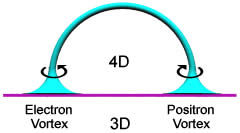Fig 7.1  An electron-positron vortex pair.

The fluid dynamic equations can therefore describe the charge pair production that is observed in high energy physics experiments.

The mapping from the quantum mechanical wave equation to the Navier-Stokes fluid dynamic equations described above is done for the two dimensional case. The fluid dynamics case requires a discontinuity in the medium for the vortexes to form, such as the water-air interface in the above example. The 3rd dimension contains the discontinuity.
However in our aether model a complete description of charge requires an additional dimension. The discontinuity for the vortexes to form is provided by the veil or membrane defining a 4D surface (see Matter section).
The complete Navier-Stokes equation would have to include four position variables, all orthogonal to one another. The complexity of fluid motion would increase considerably as there are more degrees of freedom in the 4D case. We could not only have rotation in an x-y plane but also the z-w plane at the same time, where w is the 4th axis.
Charles Hinton gives a good description of rotation in 4 dimensions .
Interestingly one of the properties of rotations in 4D space is that one requires 720 degrees rotation to bring a 4D object back to its starting point, rather than the usual 360.
This is reminiscent of quantum mechanical spin states. For example, rotating a spin-1/2 particle by 360 degrees does not bring it back to the same quantum state. To do that one needs 720 degrees rotation.
A clue perhaps that 4D space is part of our reality.

#### Particles or Waves?

One of the more ambiguous concepts of modern day Physics is the particle wave duality of matter. Particles are treated as waves under certain conditions and solid objects in others.
The basic dilemma is often illustrated by resorting to the double slit experiment involving electrons.
If electrons are fired through a double slit arrangement as shown in Fig 7.2, the probability of detecting electrons on the back plane would show a pattern that is consistent with interference effects that one observes with waves.Fig 7.2. The double slit experiment with electrons.

What is even more surprising is that if the beam intensity is reduced to such an extent that only single electrons go through the slits at any one time the same intensity pattern will appear when a large number of hits are recorded.
In other words, even when a single electron goes through the slits it behaves as if it was going through both slits and interfering with itself.
The dilemma then is that if the electrons are to be viewed as particles confined to a small region of space they can only go through one slit or the other but not both.
To resolve this dilemma Physics has come up with the notion that the electrons consist of some sort of amorphous extension in space, as described by the wave function, which moves through both slits and creates the interference pattern.
And that when we come to detect the electrons using our instruments this amorphous 'substance' somehow collapses into the localized particle. A strange notion indeed!
It leads to many conceptual difficulties and paradoxes. For example, how does the wave function know when to collapse?
The Copenhagen interpretation of quantum mechanics assumes that the wave function collapses upon observation. However the wave function doesn't know that a person is going to be reading a detector used in a measurement when it decides to collapse at the detector.
We do not think it is that clever.
What criterion should we use for the collapse of the wave function?
One might suppose that it collapses when it, or some aspect of it, encounters a 'solid' object such as a detector, but if that is so why does it not collapse when it encounters the object containing the slits?
As discussed above, we think there might be a more common sense interpretation of these events. Namely that the interference effects are not to be attributed to the electrons themselves but to the wave nature of the medium.

The above example is to a large extent academic because it would not be practical to carry out this experiment.
There are however other experiments that have been carried out that demonstrate this principle.
One common example is the scattering of electrons by a crystal, such as occurs in an electron microscope. There the scattering of electrons, or diffraction pattern, definitely displays the interference effects normally associated with waves.
However we believe that it is not necessary to attribute the wave properties to the electrons but rather to the 4D aetheric waves that surround the atoms.
The patterns of aetheric vibration will be modified by the presence of the crystalline atoms. The regular periodicity of these atoms will introduce periodic variations in the 4D standing wave patterns inside and near the crystal.
Therefore, an electron fired into the crystal would not only be affected by the Coulomb forces of the atoms but also by the 4D wave patterns.
It is conceivable that the diffraction patterns we observe in the scattered electrons are in fact due to the influence of the aetheric vibration patterns on the electrons as they pass through the crystal.
The influence being such as to produce an effect that is consistent with the observed relationship of the wavelength being proportional to the momentum of the incoming electrons.

One example of how this could work in practice is demonstrated by the Yves Coulder and Emmanuel Fort experiment  with silicon oil drops.
A droplet bouncing on a vertically vibrated silicon oil bath becomes coupled to the surface wave it generates and then moves with it. Thus we have a coupled system of particle plus circular waves generated from the bouncing.
The amazing thing is that when these particle/wave entities are sent one at a time through the equivalent of a double slit aperture they reproduce the standard interference pattern that we see with atomic particles!
See the YouTube video for a demonstration of this effect.
This has similarities to the De Broglie-Bohm pilot-wave theory which has been used to describe many of the properties at the quantum level. The theory assumes that particles are guided in their paths by some 'real' quantum wave or carrier wave existing independently of the particles. The pilot-wave can effect and be affected by the particles.
We think this approach is closer to the truth than the Copenhagen interpretation.

#### Sub-atomic Particles and Higher Dimensions

As outlined in the Matter section, the present model assumes nuclear particles to be condensations of aether at points where 4D waves produce nodes of low vibration, in a similar way that water vapour condenses into water droplets.
However we believe that in the case of nuclear particles the aetheric particles are themselves structured. That is, the aether constituting the nuclear particles forms a crystalline or ordered structure rather than a liquid form as in a water droplet.
In this model the different sub-atomic particles that Physics has discovered are a consequence of the different arrangements of the aether particles from which they are formed.

We also believe that the aether particles come in different sizes and qualities and are therefore able to form their own sub-units, somewhat analogous to the normal atoms but much smaller.
This implies that the aether particles are themselves constituted from even smaller particles in a similar way that atomic particles are formed from the aether ones.
If that were the case, then it would make sense that these sub-aether particles could be related to a 5th dimension in the same way that aether particles are related to the 4th dimension.
That is, the aether particles would be prevented from moving into the 5th dimension by some sort of veil spanning the surface of a 5D sphere, in the same way that nuclear particles are prevented from moving along the 4th dimensional axis (see "The 4th Dimension" in the Matter section).
However the sub-aether particles would be able to pass through the 5D veil and thus form vortexes or 'wormholes' channeling this rarefied matter in and out of the 4th dimension via the 5th dimension.

It doesn't take too much of a leap to imagine that there could be several levels down of particle sizes, each more rarefied and each corresponding to the next higher dimension as per the previous example.
Until a level is reached where there exists pure consciousness, as the eastern mystics suggest. Thus we can begin to understand the statements of esoteric and eastern teachings that the manifest worlds are created and maintained by consciousness. That life is a play of consciousness.

There are some similarities between the above model and the so called string theories of modern Physics. Matter is viewed as consisting of tiny strings which vibrate in different dimensions. Different modes of vibration giving rise to different particles and forces .
However these extra dimensions are assumed to wrap around themselves at a very small scale, much smaller in size than atomic particles. This is the physicist's way of explaining why we do not see these extra dimensions.
However we are suggesting here that the extra dimensions actually extend indefinitely along the axes perpendicular to the 3D ones that we are familiar with, it is just that we are prevented from moving along these.
We are also suggesting that there are a number of particle size levels below the nuclear size one, in fact 7 basic levels corresponding to 7 dimensions.
It is also interesting to note that in recent years physicists have been increasingly talking about vibrating membranes as the foundations of matter. Membranes spanning surfaces in higher dimensions, similar to the veil idea presented here .

The picture presented here is consistent with another image we get from the eastern and esoteric philosophies, that of the soul being wrapped in different sheaths or bodies.
The physical being the most dense, followed by the aetheric body which is composed of aetheric particles and so on for subsequent levels.
The esoteric teachings suggest there are 7 basic levels corresponding to the chakras or energy centers of the body. Thus each energy body forms a template for the next lower dimensional body.
The Chinese system of acupuncture for example is based on the premise that energy imbalances in the aetheric body manifest themselves in physical symptoms as disease.
This begins to make sense in the context of the present model.
Psychics have reported seeing energy vortexes corresponding to the 7 chakras of the body. There is in fact some scientific evidence for the chakra concept.
Some time ago Valerie Hunt conducted experiments at the University of California in which she used electromyographic equipment to measure low voltage waveforms from electrical activity in muscles . Electrodes were attached to the traditional chakra locations and acupuncture points of a subject. The output of the equipment was recorded together with a concurrent description of the color, size and activity of the auric fields around the subject given by an experienced aura reader. Over a number of tests there was found to be a consistent correlation between the electrical signals and the reader's aura descriptions.
The vortex nature of the chakras implies that the 3D form or body has energetic connections to all the higher bodies and higher dimensions each of which has an impact on the physical.

At death the soul leaves the physical sheath or body behind, but still retains the aetheric and the 'higher' bodies. At that point the limited consciousness of the soul is tied to the aetheric or 4D body and is unaware of the 5th dimension or higher, just as the 3D consciousness is only aware of the 3D realm.
For the soul to progress to higher levels of consciousness it has to progressively detach itself from the higher dimensional bodies, until it finally becomes free from all limitation.

It is interesting to consider a possible relationship of these seven dimensions to the ideas in string theory.
Modern string theory requires 11 dimensions to be able to describe all the forces and particles in a unified way. This includes the familiar 3 dimensions plus one time dimension, leaving us with 7 additional dimensions.
Perhaps these 7 dimensions correspond to the 7 dimensions of the higher dimensional bodies associated with the chakra centers.